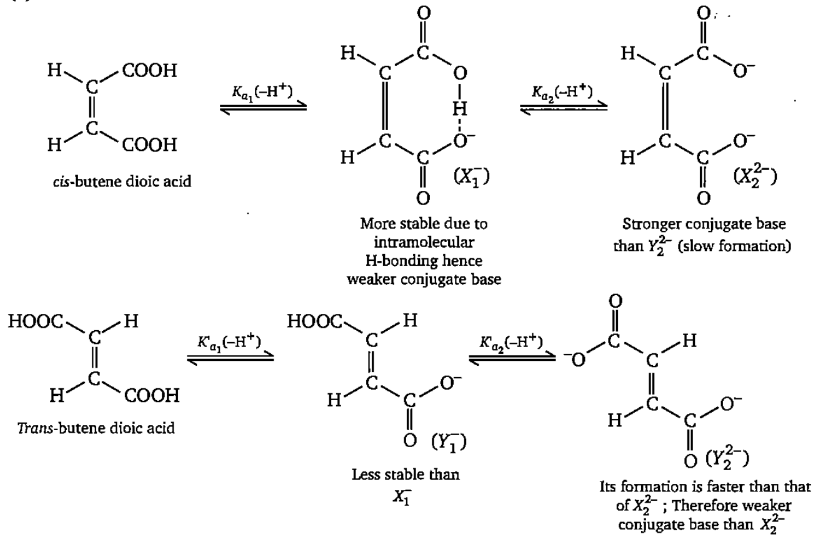Cis-butene dioic acid $\stackrel{{\mathrm{K}}_{{\mathrm{a}}_{1}}\left\{-{\mathrm{H}}^{+}\right\}}{⇌}{{\mathrm{X}}^{-}}_{1}\stackrel{{\mathrm{K}}_{{\mathrm{a}}_{2}}\left\{-{\mathrm{H}}^{+}\right\}}{⇌}{\mathrm{X}}_{2}^{2-}$

Trans-butene dioic acid $\stackrel{{\mathrm{K}}_{{\mathrm{a}}_{1}}\text{'}\left\{-{\mathrm{H}}^{+}\right\}}{⇌}{{\mathrm{Y}}^{-}}_{1}\stackrel{{\mathrm{K}}_{{\mathrm{a}}_{2}}·\left\{-{\mathrm{H}}^{+}\right\}}{⇌}{\mathrm{Y}}_{2}^{2-}$

The incorrect statement regarding above the information is:

(a) ${\mathrm{X}}_{2}^{2-}$ species is more basic than ${\mathrm{Y}}_{2}^{2-}$ species

(b) ${\mathrm{X}}_{1}^{-}$ species is more basic than ${\mathrm{Y}}_{1}^{-}$ species

(c) ${\mathrm{K}}_{\mathrm{a}1}$ is greater than $\mathrm{K}{\text{'}}_{\mathrm{a}2}$

(d) $\mathrm{K}{\text{'}}_{\mathrm{a}2}$ is greater than $\mathrm{K}{\text{'}}_{\mathrm{a}2}$

Concept Videos :-

#16 | Fajan's Rule

Concept Questions :-

Acid, Base and Fajan's Rule

(b)Difficulty Level:

• 11%
• 62%
• 22%
• 7%